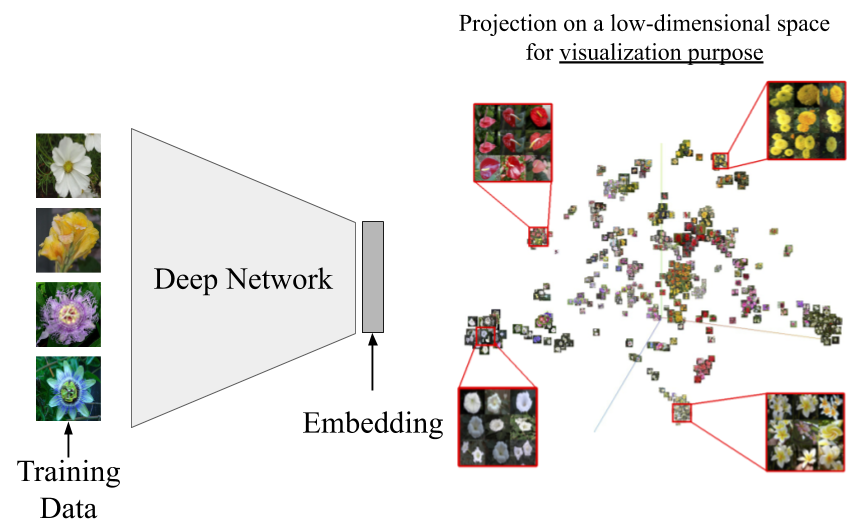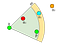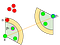# Retrieval with Deep Learning: A Ranking loss Survey Part 1

Retrieval networks are essential for searching and indexing. Deep learning leverage various ranking losses to learn an object embedding — an embedding where objects from the same class are closer than objects from different classes. This article compares various well-known ranking losses in terms of their formulations and applications. In addition, the resources section provides a TensorFlow (1.14 and 2.0) retrieval baseline for those interested in a hands-on experience.

Retrieval with deep learning is formally known as Metric learning (ML). In this learning paradigm, neural networks learn an embedding — a vector with a compact dimensionality like R^128. Such embedding quantifies the similarity between different objects as shown in the next figure. The learned embedding enables searching, nearest neighbor retrieval, indexing, etc.A deep network trained with a ranking loss to enable searching and indexing.

This survey compares various ranking losses in terms of their formulation and application. The survey is divided into two parts. This part presents the contrastive  and triplet  losses. The second part will present N-pairs  and Angular losses.

Contrastive loss 

The oldest, and simplest, ranking loss. This loss minimizes and maximizes the Euclidean distance between similar and different points, respectively. Similar and different points are grouped into positive and negative pairs. The next figure shows its formulation using a pair of points’ embeddings (x_i,x_j). y=0 when (x_i,x_j) embeddings belong to the same class. In this case, the first term minimizes the Euclidean distance D(x_i,x_j) while the second term is idle, i.e., equals zero. When the embeddings (x_i,x_j )belong to different classes, y=1, the second term maximizes the distance between the points while the first term equals zero. The max(0,m-D), in the second term, makes sure different embeddings are apart by a certain margin, i.e., a finite distance. During training, This margin makes sure the neural network’s gradient disregards abundant far (easy) negatives and leverages scarce nearby (hard) negatives.

Despite its popularity, the contrastive loss achieves humble performance in most retrieval tasks, usually used as a baseline. Most advance ranking losses require a triplet (x_i,x_j,x_k) where (x_i,x_j) belong to the same class while (x_i,x_k) belong to different classes. Such triplet sampling is difficult to acquire in unsupervised learning. Thus, despite its humble performance in retrieval, the contrastive loss is commonly used in unsupervised and self-supervised learning literature.

Triplet loss 

The most popular ranking loss is Triplet loss. It tackles an important limitation in contrastive loss’s push force. If two points are different, the contrastive loss pushes both points in the opposite direction. This solution is not optimal if one of those points is already at the center of its cluster. Triplet loss tackles this limitation using triplets instead of pairs. The triplet (x_i,x_j,x_k) are usually dubbed (anchor, positive, negative), i.e.,(a,p,n). Triplet loss pulls the anchor and positive together while pushing the anchor and negative away from each other.

Similar to the contrastive loss, the triplet loss leverage a margin m. The max and margin m make sure different points at distance > m do not contribute to the ranking loss. Triplet loss is generally superior to the contrastive loss in retrieval applications like Face recognition, Person re-identification, and feature embedding. Yet, the contrastive loss remains dominant in unsupervised learning. It is difficult to sample meaningful triplets from un-labeled data. Triplet loss seems sensitive to noisy data, so random negative sampling hinders its performance.

Triplet loss’s performance is heavily dependent on the triplet sampling strategy. Thus, there are a large number of triplet loss variants. These variants employ the same triplet loss formulation but different triplet sampling strategies. In vanilla triplet loss, triplets are randomly sampled from the training dataset. Random sampling suffers a slow convergence. Thus, a ton of papers has studied hard-mining to find useful triplets and speed convergence. The next paragraphs compare two well-known hard-mining strategies: Hard and Semi-hard sampling strategies.

In both strategies, each training mini-batch contains K*P randomly sampled training examples from K classes with P samples per class. For example, if the training batch’s size is B=32 and P=4, then the batch will contain samples from K=8 different classes, P=4 instances per class. Now, Every anchor has (P-1=3) possible positive instances and (K-1)*P=28 possible negative instances.

In hard sampling, the farthest positive and closest negative only are utilized. In the next Figure, n_3 is the closest negative for the anchor a. Thus, assuming p is the farthest positive, the loss will be computed using the triplet (a,p,n_3). This strategy converges faster because, during training, It leverages the hardest samples. However, the training hyperparameters (e.g., learning rate and batch size) need to be tuned carefully to avoid model collapse. A model collapse happens when all embedding becomes the same, i.e., f(x)=0.Triplet loss tuple (anchor, positive, negative) and margin m. Hard, semi-hard and easy negatives highlighted in red, cyan and orange respectively.

To avoid the training instability of hard-sampling, the semi-hard sampling pairs every anchor with every positive point. In the next figure, the anchor (a) will be paired with all five positives. For each positive, a negative is selected to be farther from the positive but within the banned margin m. Thus, the pair (a, p_2) will leverage the red negative inside the orange margin. This sampling strategy is more robust to the model collapse but converges slower than the hard-mining strategy.Hard sampling promotes unimodal embedding by picking the farthest positive and nearest negative (a, p1, n). Semi-hard sampling picks (a, p2, n) and avoids any tuple (a, p, n) where n lies between a and p.

Triplet loss’s sampling strategies are heavily studied in recent literature. A dedicated article is required to cover all proposed variants. The two aforementioned strategies are just the established ones — supported in the Tensorflow library. Most deep learning frameworks provide contrastive and triplet losses’ APIs. This next part of this survey will cover another two well-established ranking losses: N-pairs and Angular. Stay Tuned!

Resources

I write reviews on computer vision papers. Writing tips are welcomed.

## More from Ahmed Taha

I write reviews on computer vision papers. Writing tips are welcomed.